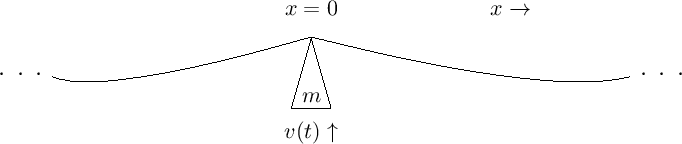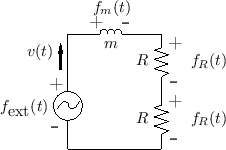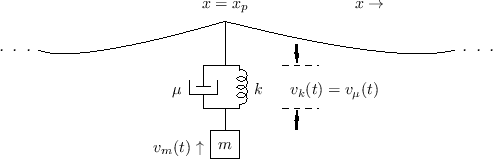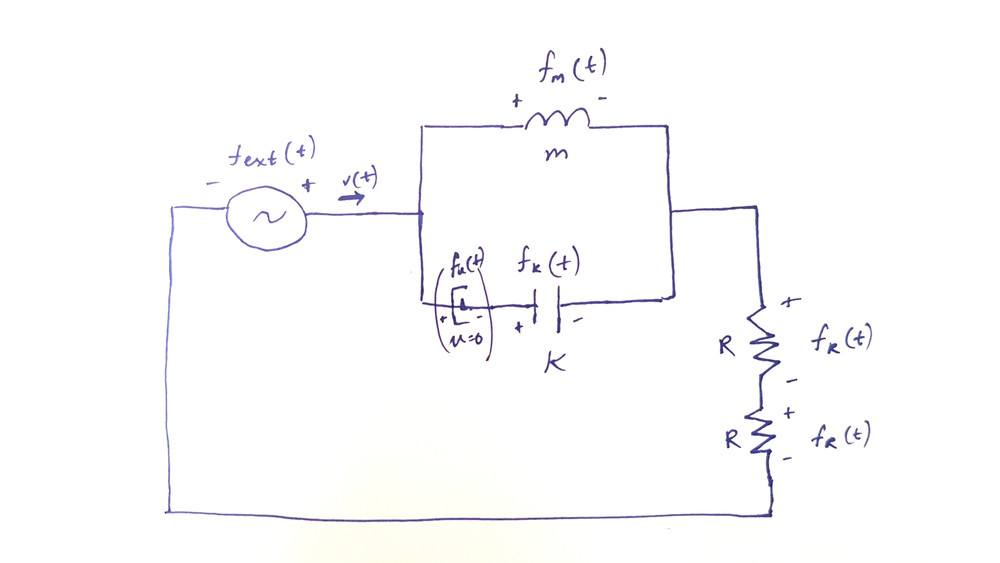# What is the electrical equivalent circuit for this interaction?

mikejm
A textbook gives an example of an ideal mass striking an ideal string here:This is drawn as an equivalent electrical circuit as follows, where each R represents one of the two string segments the mass interacts with (ie. the string segment to the left of the mass and the string segment to the right):A piano hammer is imagined slightly differently as a mass driving a damped spring against the string here (where the damping is roughly 0):They state: "The impedance of this plucking system, as seen by the string, is the parallel combination of the mass impedance ms and the damped spring impedance mu + k/s. The damper mu and spring k/s are formally in series."

I am wondering how to draw the equivalent electrical circuit for this interaction. I am trying to figure out how to write equations for the forces and velocities of a hammer modeled in this way but I think I need the diagram first.

Here is my best guess. I put the damper (which is u=0) and spring in series, and their combination in parallel to the mass. I am uncertain of the + or - directions for the damper and spring:In the first case of the mass striking a string, because all the elements were in series, I believe the sum of forces (voltages) for the elements had to equal zero (Fm(t) + Fr(t) + Fr(t) + Fext(t) = 0). What would that equation of forces look like for this circuit?

I'm guessing it would be something like an equation system based on the parallel sections:

Fm(t)+Fr(t)+Fr(t)+Fext(t)=0
Fk(t)+Fr(t)+Fr(t)+Fext(t)=0
Fm(t)=Fk(t)

Is that correct?

•